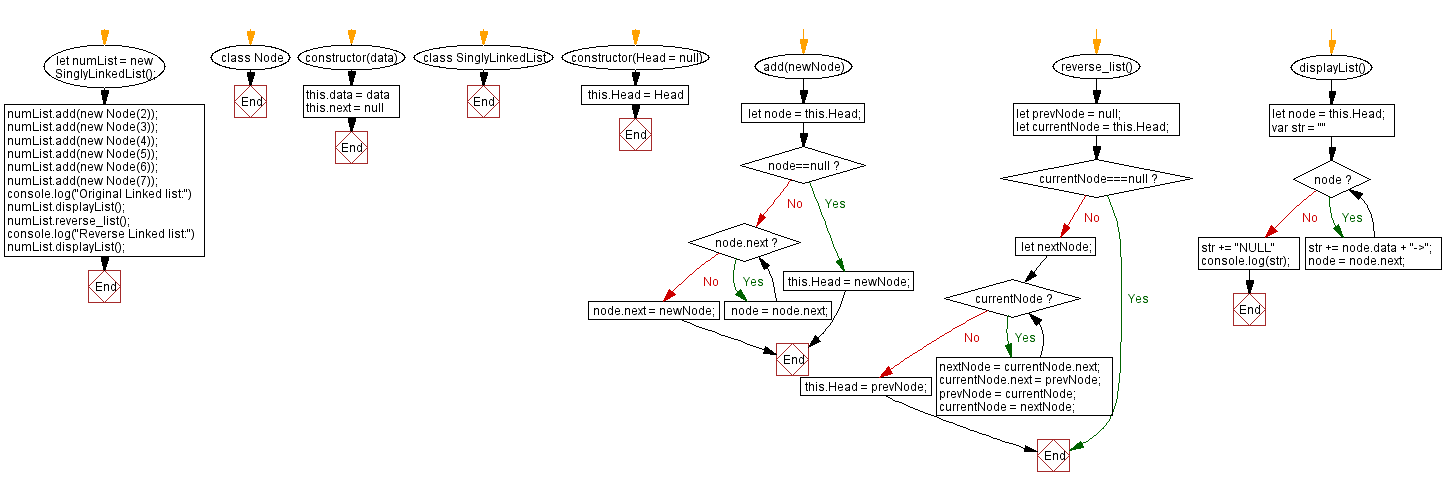# JavaScript Exercises: Print a singly linked list in reverse order

## JavaScript Singly Linked List: Exercise-2 with Solution

Write a JavaScript program to create a singly linked list of n nodes and display it in reverse order.

Sample Solution:

JavaScript Code:

``````class Node {
constructor(data) {
this.data = data
this.next = null
}
}
}
if(node==null){
return;
}
while (node.next) {
node = node.next;
}
node.next = newNode;
}

reverse_list(){
let prevNode = null;
if(currentNode===null) return;

let nextNode;
while(currentNode){
nextNode = currentNode.next;
currentNode.next = prevNode;
prevNode = currentNode;
currentNode = nextNode;
}
}

displayList(){
var str = ""
while (node) {
str += node.data + "->";
node = node.next;
}
str += "NULL"
console.log(str);
}
}
numList.displayList();
numList.reverse_list();
numList.displayList();

```
```

Sample Output:

```Original Linked list:
2->3->4->5->6->7->NULL
7->6->5->4->3->2->NULL
```

Flowchart:Live Demo:

See the Pen javascript-singly-linked-list-exercise-2 by w3resource (@w3resource) on CodePen.

Improve this sample solution and post your code through Disqus